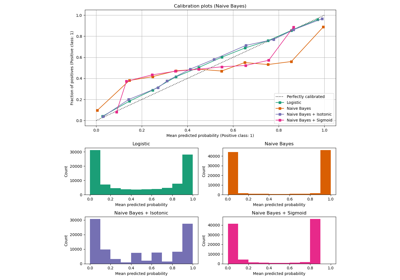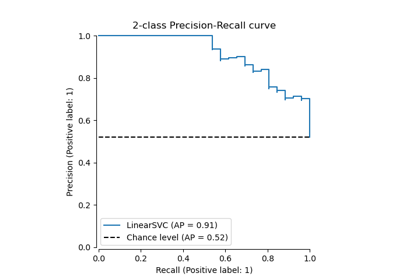# sklearn.metrics.recall_score¶

sklearn.metrics.recall_score(y_true, y_pred, *, labels=None, pos_label=1, average='binary', sample_weight=None, zero_division='warn')[source]

Compute the recall.

The recall is the ratio tp / (tp + fn) where tp is the number of true positives and fn the number of false negatives. The recall is intuitively the ability of the classifier to find all the positive samples.

The best value is 1 and the worst value is 0.

Read more in the User Guide.

Parameters:
y_true1d array-like, or label indicator array / sparse matrix

Ground truth (correct) target values.

y_pred1d array-like, or label indicator array / sparse matrix

Estimated targets as returned by a classifier.

labelsarray-like, default=None

The set of labels to include when average != 'binary', and their order if average is None. Labels present in the data can be excluded, for example to calculate a multiclass average ignoring a majority negative class, while labels not present in the data will result in 0 components in a macro average. For multilabel targets, labels are column indices. By default, all labels in y_true and y_pred are used in sorted order.

Changed in version 0.17: Parameter labels improved for multiclass problem.

pos_labelint, float, bool or str, default=1

The class to report if average='binary' and the data is binary. If the data are multiclass or multilabel, this will be ignored; setting labels=[pos_label] and average != 'binary' will report scores for that label only.

average{‘micro’, ‘macro’, ‘samples’, ‘weighted’, ‘binary’} or None, default=’binary’

This parameter is required for multiclass/multilabel targets. If None, the scores for each class are returned. Otherwise, this determines the type of averaging performed on the data:

'binary':

Only report results for the class specified by pos_label. This is applicable only if targets (y_{true,pred}) are binary.

'micro':

Calculate metrics globally by counting the total true positives, false negatives and false positives.

'macro':

Calculate metrics for each label, and find their unweighted mean. This does not take label imbalance into account.

'weighted':

Calculate metrics for each label, and find their average weighted by support (the number of true instances for each label). This alters ‘macro’ to account for label imbalance; it can result in an F-score that is not between precision and recall. Weighted recall is equal to accuracy.

'samples':

Calculate metrics for each instance, and find their average (only meaningful for multilabel classification where this differs from accuracy_score).

sample_weightarray-like of shape (n_samples,), default=None

Sample weights.

zero_division{“warn”, 0.0, 1.0, np.nan}, default=”warn”

Sets the value to return when there is a zero division.

Notes: - If set to “warn”, this acts like 0, but a warning is also raised. - If set to np.nan, such values will be excluded from the average.

New in version 1.3: np.nan option was added.

Returns:
recallfloat (if average is not None) or array of float of shape (n_unique_labels,)

Recall of the positive class in binary classification or weighted average of the recall of each class for the multiclass task.

precision_recall_fscore_support

Compute precision, recall, F-measure and support for each class.

precision_score

Compute the ratio tp / (tp + fp) where tp is the number of true positives and fp the number of false positives.

balanced_accuracy_score

Compute balanced accuracy to deal with imbalanced datasets.

multilabel_confusion_matrix

Compute a confusion matrix for each class or sample.

PrecisionRecallDisplay.from_estimator

Plot precision-recall curve given an estimator and some data.

PrecisionRecallDisplay.from_predictions

Plot precision-recall curve given binary class predictions.

Notes

When true positive + false negative == 0, recall returns 0 and raises UndefinedMetricWarning. This behavior can be modified with zero_division.

Examples

>>> import numpy as np
>>> from sklearn.metrics import recall_score
>>> y_true = [0, 1, 2, 0, 1, 2]
>>> y_pred = [0, 2, 1, 0, 0, 1]
>>> recall_score(y_true, y_pred, average='macro')
0.33...
>>> recall_score(y_true, y_pred, average='micro')
0.33...
>>> recall_score(y_true, y_pred, average='weighted')
0.33...
>>> recall_score(y_true, y_pred, average=None)
array([1., 0., 0.])
>>> y_true = [0, 0, 0, 0, 0, 0]
>>> recall_score(y_true, y_pred, average=None)
array([0.5, 0. , 0. ])
>>> recall_score(y_true, y_pred, average=None, zero_division=1)
array([0.5, 1. , 1. ])
>>> recall_score(y_true, y_pred, average=None, zero_division=np.nan)
array([0.5, nan, nan])

>>> # multilabel classification
>>> y_true = [[0, 0, 0], [1, 1, 1], [0, 1, 1]]
>>> y_pred = [[0, 0, 0], [1, 1, 1], [1, 1, 0]]
>>> recall_score(y_true, y_pred, average=None)
array([1. , 1. , 0.5])


## Examples using sklearn.metrics.recall_score¶Probability Calibration curves

Probability Calibration curvesPrecision-Recall

Precision-Recall